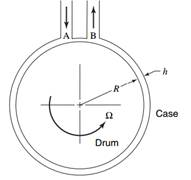### Create an Account

Already have account?

### Forgot Your Password ?

Home / Questions / For the flow described in Problem 813 obtain the differential equation of motion if vθ frt...

# For the flow described in Problem 813 obtain the differential equation of motion if vθ frt Problem 813 The device in the schematic diagram below is a viscosity pump. It consists of

For the flow described in Problem 8.13, obtain the differential equation of motion if vθ = f(r; t).

Problem 8.13

The device in the schematic diagram below is a viscosity pump. It consists of a rotating drum inside of a stationary case. The case and the drum are concentric. Fluid enters at A, flows through the annulus between the case and the drum, and leaves at B. The pressure at B is higher than that at A, the difference being ∆ρ: The length of the annulus is L. The width of the annulus h is very small compared to the diameter of the drum, so that the flow in the annulus is equivalent to the flow between two flat plates. Assume the flow to be laminar. Find the pressure rise and efficiency as a function of the flow rate per unit depth.2. Determine the velocity profile in a fluid situated between two coaxial rotating cylinders. Let the inner cylinder have radius R1, and angular velocity Ω1; let the outer cylinder have radius R2 and angular velocity Ω2.

Mar 28 2020 View more View Less

#### Answer (Solved)Subscribe To Get Solution